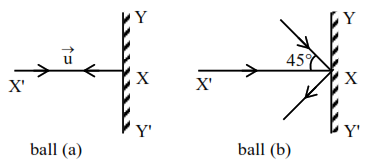# Two billiard balls of equal mass`
Question:

Two billiard balls of equal mass $30 \mathrm{~g}$ strike a rigid wall with same speed of $108 \mathrm{kmph}$ (as shown) but at different angles. If the balls get reflected with the same speed then the ratio of the magnitude of impulses imparted to ball ' $a$ ' and ball ' $b$ ' by the wall along ' $X$ ' direction is :1. $1: 1$

2. $\sqrt{2}: 1$

3. $2: 1$

4. $1: \sqrt{2}$

Correct Option: , 2

Solution:

Impulse $=$ change in momentum

Ball (a) $|\overrightarrow{\Delta \mathrm{p}}|=2 \mathrm{mu}=\mathrm{J}_{1}$

Ball (b) $|\overrightarrow{\Delta \mathrm{p}}|=2 \mathrm{mu} \cos 45^{\circ}=\mathrm{J}_{2}$

$\frac{J_{1}}{J_{2}}=\frac{1}{\cos 45^{\circ}}=\sqrt{2}$